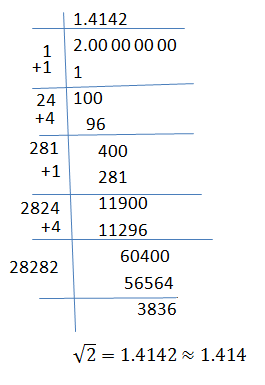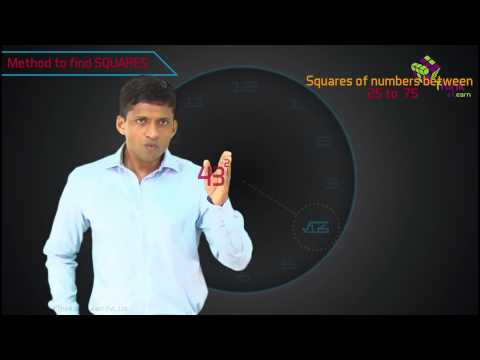# Square Root Questions

Solving square root questions will help you to get handy with squares and square roots of the numbers. It has been often said that “practice makes perfect.” This phrase is very relatable when it comes to math as students can be good at the subject only by practising and solving problems more and more. While it may not be easy, but developing math skills and fluency is important. Mathematical skills of investigation, problem-solving, and logical thinking can further help students progress in other subjects as well.

Here we will be discussing the topic of square roots. Well, generally when we define the term, the square root of a number is a value that, when multiplied by itself, gives the number. For example, let’s say when you multiply 4 × 4 you get 16. So the square root of 16 is 4.

The symbol denotes it is √ , and it means that it is a positive or perfect square root. For example, √36 = 6 (6 × 6 = 36). There are also negative square numbers. For example, (-5) × (-5) = 25. When we square a negative number, we get a positive result.

Moving on, if you want to know how to find the square root of a number, then there are a lot of methods. However, the most basic method that can be used is the prime factorization method or the popular square root long division method. You can use this method to find the square root of a number that is satisfactory or accurate enough for you. An example of the division method showing how to take the square root is given below;Additionally, you can also check out BYJU’S YouTube channel to learn how to find the square of any number using different methods.

## Perfect Square Root Questions

Here are some multiple-choice questions based on perfect square numbers. Solve them and find the answers. The answers to these questions are also provided at the end to crosscheck.

1. Which of the following numbers is a perfect square?

(a) 141

(b) 196

(c) 124

(d) 222

2. A perfect square number can never have the digit ….. at the units place.

(a) 1

(b) 4

(c) 8

(d) 9

3. Evaluate √6084

(a) 75

(b) 77

(c) 78

(d) 68

4. Find the square root of 5929.

(a) 49

(b) 33

(c) 77

(d) 73

5. Evaluate √1471369.

(a) 1213

(b) 1223

(c) 1233

(d) 1243

1 – (b), 2 – (c), 3 – (c), 4 – (c), 5 – (a)

Also, find:

### Square Root Question for Class 8

Q.1 Find the square root of 125.

Q.2 Find the square root of 122 by long division method.

Q.3 Find the square root of 225 by prime factorisation method.

Q.4: Is 2352 a perfect square? If not, find the smallest multiple of 2352 which is a perfect square. Find the square root of the new number.

Q.5: Find the smallest square number which is divisible by each of the numbers 6, 9 and 15.

Use the table below for squares and the square root of the number from 1 to 15 to solve the questions based on the square root.

Square Root Table

 Number Squares Square Root (Upto 3 places of decimal) 1 12 = 1 √1 = 1 2 22 = 4 √2 = 1.414 3 32 = 9 √3 = 1.732 4 42 = 16 √4 = 2.000 5 52 = 25 √5 = 2.236 6 62 = 36 √6 = 2.449 7 72 = 49 √7 = 2.646 8 82 = 64 √8 = 2.828 9 92 = 81 √9 = 3.000 10 102 = 100 √10 = 3.162` 11 112 = 121 √11 = 3.317 12 122 = 144 √12 = 3.464 13 132 = 169 √13 = 3.606 14 142 = 196 √14 = 3.742 15 152 = 225 √15 = 3.873